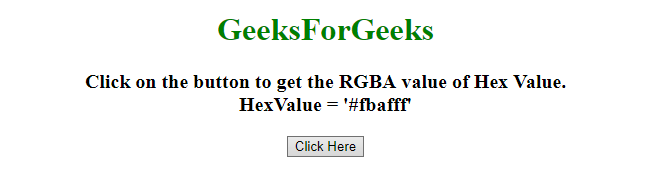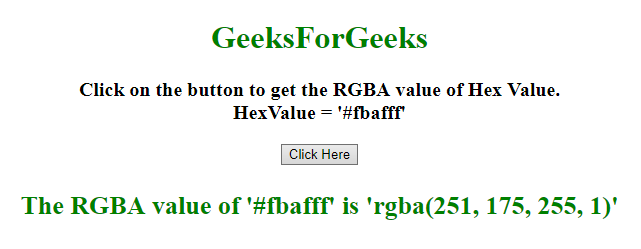# How to convert Hex to RGBA value using JavaScript ?

• Last Updated : 31 Dec, 2019

Given a color hex code and the task is to convert Hex Value to RGBA value. Here are few of the mostly techniques discussed with the help of JavaScript.

Approach 1:

Hey geek! The constant emerging technologies in the world of web development always keeps the excitement for this subject through the roof. But before you tackle the big projects, we suggest you start by learning the basics. Kickstart your web development journey by learning JS concepts with our JavaScript Course. Now at it's lowest price ever!

• First check the passed Hex value is valid or not through a Regular Expression.
• Then get the hex value content after ‘#’ by using .slice() method and use .split() method to get the every character of the hex value in the array.
• If the length of array is 3(eg. #fff, #aaa) then store them as(eg. #ffffff, #aaaaaa respectively) to make the length 6.
• Transform the code to Hexadecimal as first 2 character belongs to Red Value, mid 2 character belongs to Green value and so on.

Example: This example uses the approach discussed above.

 ` ``<``html``> `` ` `<``head``> ``    ``<``title``> ``        ``How to convert Hex value to RGBA``        ``value using JavaScript``    ```` `` ` `<``body` `style` `= ``"text-align:center;"``> ``     ` `    ``<``h1` `style` `= ``"color: green"``> ``        ``GeeksForGeeks ``    ````     ` `    ``<``p` `id` `= ``"GFG_UP"` `style` `=``        ``"font-size: 20px; font-weight: bold;"``>``    ````     ` `    ``<``button` `onclick` `= ``"gfg_Run()"``> ``        ``Click Here``    ````     ` `    ``<``p` `id` `= ``"GFG_DOWN"` `style = "color:green;``        ``font-size: 26px; font-weight: bold;">``    ````     ` `    ``<``script``>``        ``var el_up = document.getElementById("GFG_UP");``        ``var el_down = document.getElementById("GFG_DOWN");``        ``var hexValue = '#fbafff';``         ` `        ``el_up.innerHTML = "Click on the button to"``                ``+ " get the RGBA value of Hex "``                ``+ "Value.<``br``>HexValue = '" + ``                ``hexValue + "'";``         ` `        ``function convertHexToRgbA(hexVal) {``            ``var ret;``             ` `            ``// If the hex value is valid.``            ``if(/^#([A-Fa-f0-9]{3}){1,2}\$/.test(hexVal)) {``                 ` `                ``// Getting the content after '#',``                ``// eg. 'ffffff' in case of '#ffffff'``                ``ret = hexVal.slice(1);``                 ` `                ``// Splitting each character``                ``ret = ret.split('');``                 ` `                ``// Checking if the length is 3``                ``// then make that 6``                ``if(ret.length == 3) {``                    ``var ar = [];``                    ``ar.push(ret); ``                    ``ar.push(ret);``                    ``ar.push(ret);``                    ``ar.push(ret);``                    ``ar.push(ret);``                    ``ar.push(ret);``                    ``ret = ar;``                ``}``                 ` `                ``// Starts with '0x'(in hexadecimal)``                ``ret = '0x'+ ret.join('');``                 ` `                ``// Converting the first 2 characters``                ``// from hexadecimal to r value``                ``var r = (ret>>16)&255;``                 ` `                ``// Converting the second 2 characters``                ``// to hexadecimal to g value``                ``var g = (ret>>8)&255;``                 ` `                ``// Converting the last 2 characters``                ``// to hexadecimal to b value``                ``var b = ret&255;``                 ` `                ``// Appending all of them to make``                ``// the RGBA value``                ``return 'rgba('+[r, g, b].join(',')+',1)';``            ``}``        ``}``        ``function gfg_Run() {``            ``el_down.innerHTML = "The RGBA value of '"``                ``+ hexValue + "' is '" + ``                ``convertHexToRgbA(hexValue) + "'";``        ``} ``    `` `` `` ` ``

Output:

• Before clicking on the button:• After clicking on the button:Approach 2:

• Use .slice() method to get the first 2 characters and convert them to Hexadecimal.
• Repeat the step 1 for every 2 characters for rest of the code.
• Append them together and return the final value.

Example: This example uses the approach discussed above.

 ` ``<``html``> `` ` `<``head``> ``    ``<``title``> ``        ``How to convert Hex value to RGBA``        ``value using JavaScript ?``    ````     ` `    ``<``script` `src``=``"https://ajax.googleapis.com/ajax/libs/jquery/3.4.1/jquery.min.js"``>``    ```` `` ` `<``body` `style` `= ``"text-align:center;"``> ``     ` `    ``<``h1` `style` `= ``"color: green"``> ``        ``GeeksForGeeks ``    ````     ` `    ``<``p` `id` `= ``"GFG_UP"` `style` `= ``        ``"font-size: 20px; font-weight: bold;"``>``    ````     ` `    ``<``button` `onclick` `= ``"gfg_Run()"``> ``        ``Click Here``    ````     ` `    ``<``p` `id` `= ``"GFG_DOWN"` `style = "color:green;``        ``font-size: 26px; font-weight: bold;">``    ````     ` `    ``<``script``>``        ``var el_up = document.getElementById("GFG_UP");``        ``var el_down = document.getElementById("GFG_DOWN");``        ``var hexValue = '#fbafff';``         ` `        ``el_up.innerHTML = "Click on the button to get"``                ``+ " the RGBA value of Hex Value."``                ``+ "<``br``>HexValue = '" + hexValue + "'";``         ` `        ``function convertHexToRgbA(hex, a) {``             ` `            ``// Convert the first 2 characters to hexadecimal``            ``var r = parseInt(hex.substring(1, 3), 16),``             ` `            ``// Convert the middle 2 characters to hexadecimal``            ``g = parseInt(hex.substring(3, 5), 16),``                 ` `            ``// Convert the last 2 characters to hexadecimal``            ``b = parseInt(hex.substring(5, 7), 16);``                 ` `            ``// append them all``            ``return "rgba(" + r + ", " + g + ", "``                    ``+ b + ", " + a + ")";``        ``}``         ` `        ``function gfg_Run() {``            ``el_down.innerHTML = "The RGBA value of '"``                    ``+ hexValue + "' is '" + ``                    ``convertHexToRgbA(hexValue, 1) + "'";``        ``} ``    `` `` `` ` ``

Output:

• Before clicking on the button:• After clicking on the button:My Personal Notes arrow_drop_up